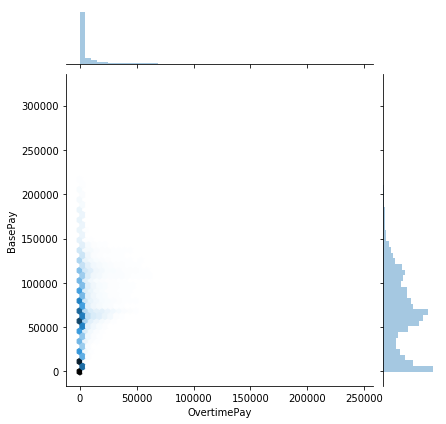** What is the average BasePay ?**

** What is the highest amount of OvertimePay in the dataset ? **

** How much does JOSEPH DRISCOLL make (including benefits)? **

** What is the name of highest paid person (including benefits)?**

** What is the name of lowest paid person (including benefits)?

** What was the average (mean) BasePay of all employees per year? (2011-2014) ? ** show the same in a plot with year in the x-axis and the average BasePay in the y-axis

** How many unique job titles are there? **

** What are the top 5 most common jobs? **

** How many Job Titles were represented by only one person in 2013? **

** create a jointplot between BasePay and OvertimePay to see if there is any correlation **

In :
``````import pandas as pd
import numpy as np``````
In :
``import seaborn as sns``
In :
``data = pd.read_csv("Salaries.csv")``
In :
``data``
Out:
In :
``````#What is the average BasePay?
data["BasePay"].mean()``````
Out:
``66325.44884050643``
In :
``````#What is the highest amount of OvertimePay in the dataset ?
data["OvertimePay"].max()``````
Out:
``245131.88``
In :
``````#How much does JOSEPH DRISCOLL make (including benefits)?
data[data["EmployeeName"]=="JOSEPH DRISCOLL"]["TotalPayBenefits"]``````
Out:
``````24    270324.91
Name: TotalPayBenefits, dtype: float64``````
In :
``````#What is the name of highest paid person (including benefits)?
max_benefit_value = data["TotalPayBenefits"].max()
data[data["TotalPayBenefits"]==max_benefit_value]["EmployeeName"]``````
Out:
``````0    NATHANIEL FORD
Name: EmployeeName, dtype: object``````
In :
``````#What is the name of lowest paid person (including benefits)
min_benefit_value = data["TotalPayBenefits"].min()
data[data["TotalPayBenefits"]==min_benefit_value]["EmployeeName"]``````
Out:
``````148653    Joe Lopez
Name: EmployeeName, dtype: object``````
In :
``````#How many unique job titles are there?
data["JobTitle"].nunique()``````
Out:
``2159``
In :
``````#create a jointplot between BasePay and OvertimePay to see if there is any correlation?
sns.jointplot(x="OvertimePay", y="BasePay", data=data, kind="hex")``````
Out:
``<seaborn.axisgrid.JointGrid at 0x134f0a07e10>``In :
``````#What are the top 5 most common jobs?
data["JobTitle"].value_counts().head()``````
Out:
``````Transit Operator                7036
Special Nurse                   4389
Registered Nurse                3736
Public Svc Aide-Public Works    2518
Police Officer 3                2421
Name: JobTitle, dtype: int64``````
In :
``data["NewCol"] = (data["BasePay"]/data["TotalPay"])*100``
In :
``data.head()``
Out:
In [ ]:
``````#How many Job Titles were represented by only one person in 2013?
data(data["year"]==2013)["JobTitle"]``````
In :
``import jovian``
In [ ]:
``jovian.commit()``
```[jovian] Saving notebook.. ```
In [ ]:
`` ``